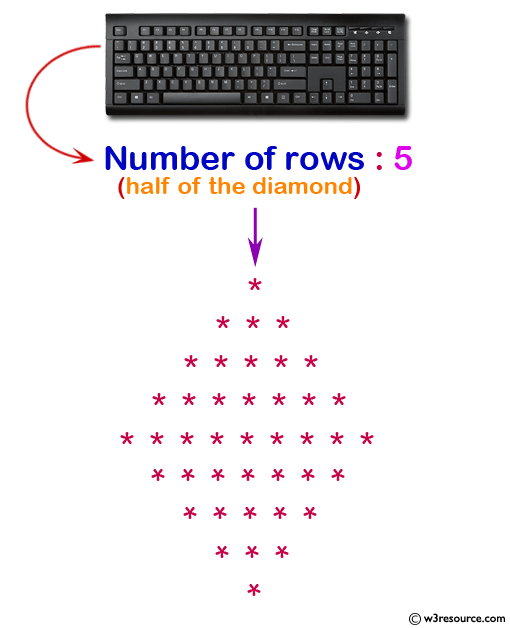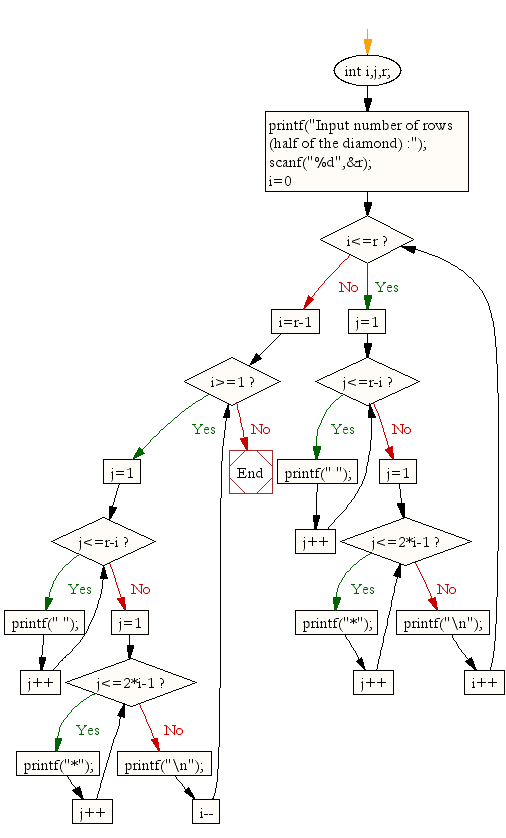﻿ C Program: Display the pattern like a diamond - w3resource# C Exercises: Display the pattern like a diamond

## C For Loop: Exercise-31 with Solution

Write a program in C to display the pattern like a diamond. The pattern is as follows :

```    *
***
*****
*******
*********
*******
*****
***
*
```

Pictorial Presentation:Sample Solution:

C Code:

``````#include <stdio.h>

void main()
{
int i,j,r;
printf("Input number of rows (half of the diamond) :");
scanf("%d",&r);
for(i=0;i<=r;i++)
{
for(j=1;j<=r-i;j++)
printf(" ");
for(j=1;j<=2*i-1;j++)
printf("*");
printf("\n");
}

for(i=r-1;i>=1;i--)
{
for(j=1;j<=r-i;j++)
printf(" ");
for(j=1;j<=2*i-1;j++)
printf("*");
printf("\n");
}

}
```
```

Sample Output:

```Input number of rows (half of the diamond) :5

*
***
*****
*******
*********
*******
*****
***
*
```

Flowchart:C Programming Code Editor:

Improve this sample solution and post your code through Disqus.

What is the difficulty level of this exercise?

Test your Programming skills with w3resource's quiz.

﻿

## C Programming: Tips of the Day

Is there a way to specify how many characters of a string to print out using printf()?

The basic way is:

`printf ("Here are the first 8 chars: %.8s\n", "A string that is more than 8 chars");`

The other, often more useful, way is:

`printf ("Here are the first %d chars: %.*s\n", 8, 8, "A string that is more than 8 chars");`

Here, you specify the length as an int argument to printf(), which treats the '*' in the format as a request to get the length from an argument.

You can also use the notation:

```printf ("Here are the first 8 chars: %*.*s\n",
8, 8, "A string that is more than 8 chars");```

This is also analogous to the "%8.8s" notation, but again allows you to specify the minimum and maximum lengths at runtime - more realistically in a scenario like:

`printf("Data: %*.*s Other info: %d\n", minlen, maxlen, string, info);`

The POSIX specification for printf() defines these mechanism

Ref : https://bit.ly/3u32GyO